In this article, we will share MP Board Class 10th Maths Book Solutions Chapter 14 Statistics Ex 14.3 Pdf, These solutions are solved subject experts from the latest edition books.

## MP Board Class 10th Maths Solutions Chapter 14 Statistics Ex 14.3

Question 1.
The following frequency distribution gives the monthly consumption of electricity of 68 consumers of a locality. Find the median, mean and mode of the data and compare them.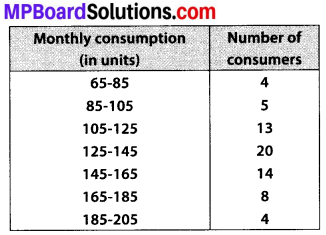Solution:
Median:
Let us prepare a cumulative frequency table: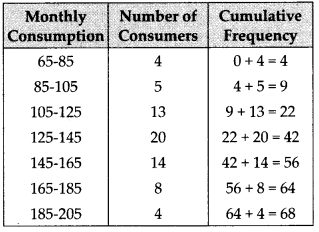Now, we have N = 68 ⇒ $$\frac{N}{2}=\frac{68}{2}$$ = 34
The cumulative frequency just greater than 34 is 42 and it corresponds to the class 125 – 145.
∴ 125 – 145 is the median class.
∴ l = 125, cf = 22, f= 20 and h = 20
Using the formula,
Median = l + $$\left[\frac{\frac{N}{2}-c f}{f}\right]$$ × h
= 125 + $$\left[\frac{34-22}{20}\right]$$ × 20
= 125 + $$\frac{12}{20}$$ × 20 = 125 + 12 = 137 units.
Mean: Let assumed mean, a = 135
∵ Class size, h = 20
∴ ui = $$\frac{x_{i}-a}{h}=\frac{x_{i}-135}{20}$$
Now, we have the following table: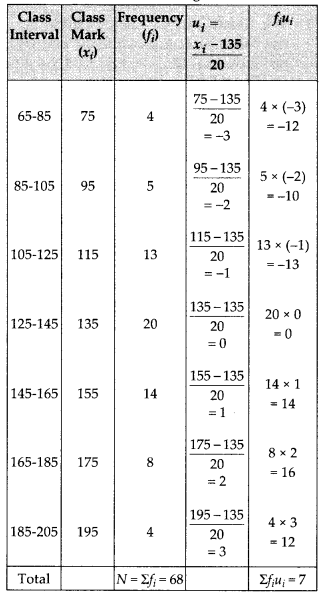∴ $$\overline{x}$$ = a + h × [$$\frac{1}{N}$$ Σfiui] = 135 + 20 × $$\frac{7}{68}$$
= 135 + 2.05 = 137.05 units.
Mode:
∵ Class 125 – 145 has the highest frequency i.e., 20.
∴ 125 – 145 is the modal class.
We have: h = 20, l = 125 , f1 = 20, f0 = 13, f2 = 14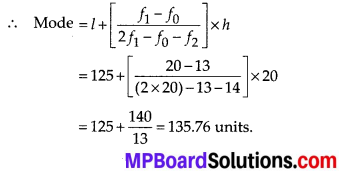We observe that the three measures are approximately equal.

Question 2.
If the median of the distribution given below is 28.5, find the values of x and y.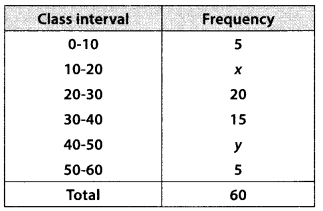Solution:
Here, we have N = 60
Now, cumulative frequency table is: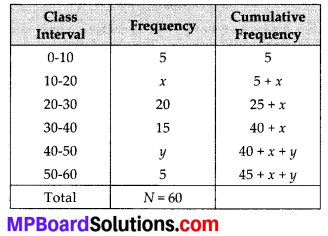Since, median = 28.5 (Given)
∴ Median class is 20 – 30 and l = 20, f = 20, cf = 5 + x, N = 60
∴ l + $$\left[\frac{\frac{N}{2}-c f}{f}\right]$$ × h
⇒ 28.5 = 20 + $$\left[\frac{30-(5+x)}{20}\right]$$ × 10
⇒ 28.5 = 20 + $$\frac{25-x}{2}$$
⇒ 57 = 40 + 25 – x
⇒ x = 40 + 25 – 57 = 8
Also, 45 + x + y = 60
⇒ 45 + 8 + y = 60
⇒ y = 60 – 45 – 8 = 7.
Thus x = 8, y = 7Question 3.
A life insurance agent found the following data for distribution of ages of 100 policy holders. Calculate the median age, if policies are given only to persons having age 18 years onwards but less than 60 year.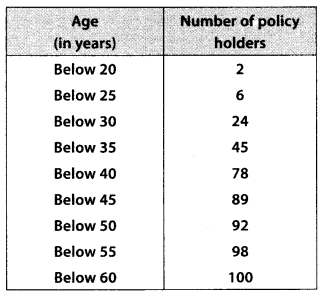Solution:
The given table is cumulative frequency distribution. We write the frequency distribution as given below :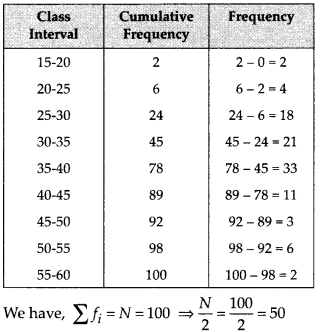∵ The cumulative frequency just greater than 50 is 78.
∴ The median class is 35 – 40.
Now, $$\frac{N}{2}$$ = 50, l = 35, cf = 45, f = 33 and h = 5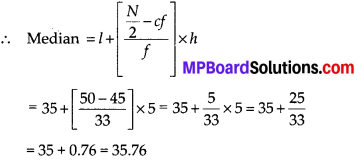Thus, the median age = 35.76 years.Question 4.
The lengths of 40 leaves of a plant are measured correct to the nearest millimeter, and the data obtained is represented in the following table: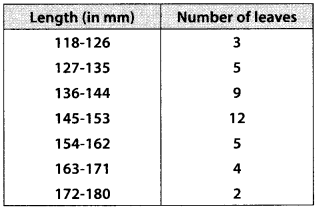Find the median length of the leaves.
[Hint: The data needs to be converted to continuous classes for finding the median, since the formula assumes continuous classes. The classes then change to 117.5-126.5,
126.5 – 135.5 ………… 171.5 – 180.5.]
Solution:
After changing the given table as continuous classes we prepare the cumulative frequency table as follows: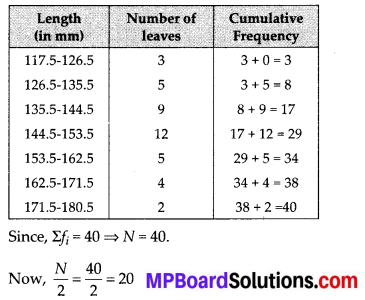The cumulative frequency just above 20 is 29 and it corresponds to the class 144.5 – 153.5.
So, 144.5 – 153.5 is the median class.
We have: $$\frac{N}{2}$$ = 20, l = 144.5, f= 12, cf = 17 and h = 9
∴ Median = l + $$\left[\frac{\frac{N}{2}-c f}{f}\right]$$ × h
= 144.5 + $$\left[\frac{20-17}{12}\right]$$ × 9
= 144.5 + $$\frac{3}{12}$$ × 9 = 144.5 + $$\frac{9}{4}$$
= 144.5 + 2.25 = 146.75
Median length of leaves = 146.75 mm.

Question 5.
The following table gives the distribution of the life time of 400 neon lamps: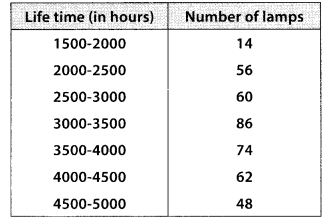Find the median life time of a lamp.
Solution:
To compute the median, let us write the cumulative frequency distribution as given below: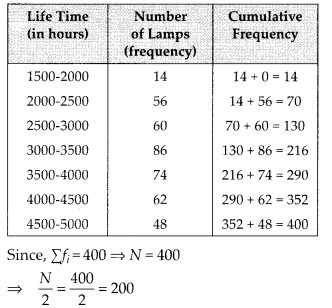Since, the cumulative frequency just greater than 200 is 216.
∴ The median class is 3000-3500 and so l = 3000, cf= 130, f = 86, h = 500
∴ Median = l + $$\left[\frac{\frac{N}{2}-c f}{f}\right]$$ × h
= 3000 + $$\left[\frac{200-130}{86}\right]$$ × 500
= 3000 + $$\frac{70}{86}$$ × 500 = 3000 + $$\frac{35000}{86}$$
= 3000 + 406.98 = 3406.98
Thus, median life time of a lamp = 3406.98 hours.Question 6.
100 surnames were randomly picked up from a local telephone directory and the frequency distribution of the number of letters in the English alphabets in the surnames was obtained as follows: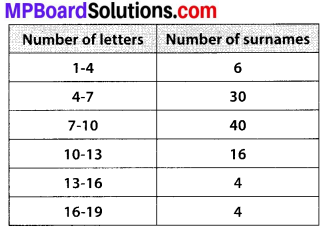Determine the median number of letters in the surnames. Find the mean number of letters in the surnames? Also, find the modal size of the surnames.
Solution:
Median: The cumulative frequency distribution table is as follows: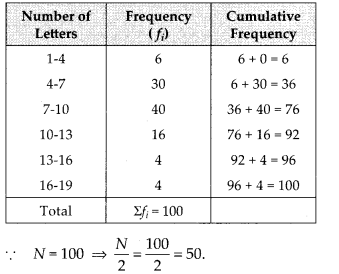Since, the cumulative frequency just greater than 50 is 76.
∴ The class 7-10 is the median class.
We have, $$\frac{N}{2}$$ = 50 , f = 7, cf = 36, f = 40 and h = 3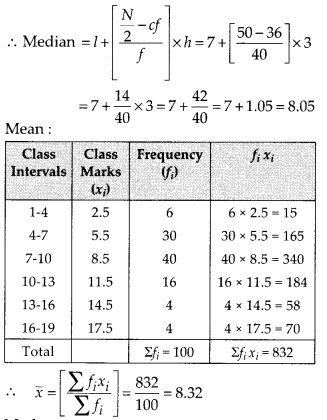Mode:
Since the class 7 – 10 has the maximum frequency i.e., 40.
∴ The modal class is 7 – 10.
So, we have l = 7,h = 3, f1 = 40, f0 = 30, f2 = 16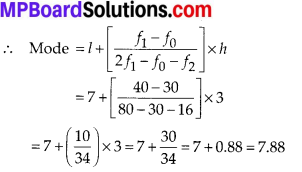Thus, the required median = 8.05, mean = 8.32 and mode = 7.88.

Question 7.
The distribution below gives the weights of 30 students of a class. Find the median weight of the students.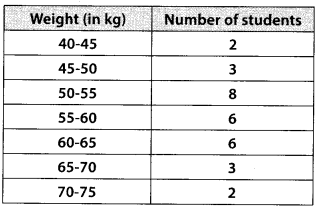Solution:
We have cumulative frequency table as follows: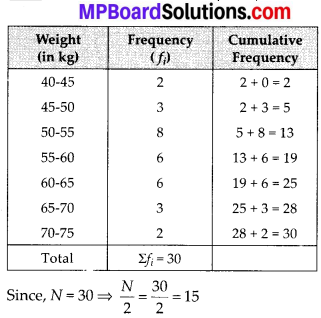The cumulative frequency just greater than 15 is 19, which corresponds to the class 55 – 60.
So, median class is 55-60 and we have $$\frac{N}{2}$$ = 15,
l = 55, f = 6, cf = 13 and h = 5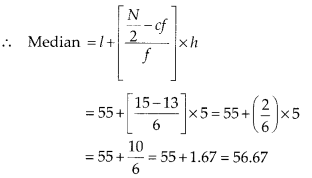Thus, the required median weight of the students = 56.67 kg.